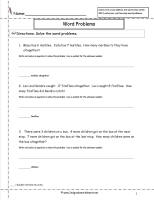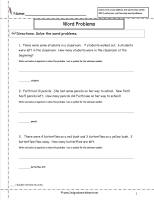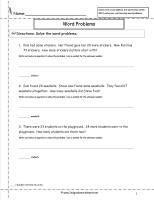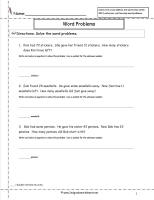﻿ CCSS 2.OA.1 Worksheets. Addition and Subtraction Word Problems Worksheets

# Common Core State Standard 2.OA.1

## Operations & Algebraic Thinking

### Represent and solve problems involving addition and subtraction.

1. Use addition and subtraction within 100 to solve one- and two-step word problems involving situations of adding to, taking from, putting together, taking apart, and comparing, with unknowns in all positions, e.g., by using drawings and equations with a symbol for the unknown number to represent the problem.

###One Digit Addition Word Problems2

Common Core State Standards: 2.OA.1
Operations & Algebraic Thinking
Represent and solve problems involving addition and subtraction.

One digit addition word problems with 1 and 2 step problems.

###One Digit Subtraction Word Problems2

Common Core State Standards: 2.OA.1
Operations & Algebraic Thinking
Represent and solve problems involving addition and subtraction.

One digit subtraction word problems with 1 and 2 step problems.

###Two Digit Addition Word Problems2

Common Core State Standards: 2.OA.1
Operations & Algebraic Thinking
Represent and solve problems involving addition and subtraction.

Two digit addition word problems with 1 and 2 step problems. No regrouping.

###Two Digit Subtraction Word Problems2

Common Core State Standards: 2.OA.1
Operations & Algebraic Thinking
Represent and solve problems involving addition and subtraction.

Two Digit Subtraction word problems with 1 and 2 step problems. No regrouping.